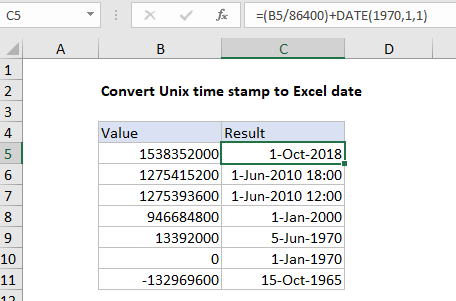## Excel Office

Excel How Tos, Tutorials, Tips & Tricks, Shortcuts

# Convert Unix time stamp to Excel date

This tutorial show how to Convert Unix time stamp to Excel date using the example below.

To convert a time in Excel’s format to a Unix time stamp, you can use a formula based on the DATE function.

## Formula

`=(A1/86400)+DATE(1970,1,1)`## Explanation of how this formula works

In the example shown, the formula in C5 is:

`=(B5/86400)+DATE(1970,1,1)`

The Unix time stamp tracks time as a running count of seconds. The count begins at the “Unix Epoch” on January 1st, 1970, so a Unix time stamp is simply the total seconds between any given date and the Unix Epoch. Since a day contains 86400 seconds (24 hours x 60 minutes x 60 seconds), conversion to Excel time can be done by dividing days by 86400 and adding the date value for January 1st, 1970.

Worked Example:   How to calculate nth day of year in Excel

In the example shown, the formula first divides the time stamp value in B5 by 86400, then adds the date value for the Unix Epoch, January 1, 1970. The formula evaluates like this:

```=(B5/86400)+DATE(1970,1,1)
=(1538352000/86400)+25569
=43374```

When C5 is formatted with the Excel date “d-mmm-yyyy”, the date is displayed as 1-Oct-2018.

Worked Example:   Add years to date in Excel

### How Excel tracks dates time

The Excel date system starts on January 1, 1900 and counts forward. The table below shows the numeric values associated with a few random dates:

Date Raw value
1-Jan-1900 1
28-Jul-1914 00:00 5323
1-Jan-1970 00:00 25569
31-Dec-1999 36525
1-Oct-2018 43374
1-Oct-2018 12:00 PM 43374.5

Notice the last date includes a time as well. Since one day equals 1, and one day equals 24 hours, time in Excel can represented as fractional values of 1, as shown in the table below. In order to see the value displayed as a time, a time format needs to be applied.

Worked Example:   Convert Excel time to Unix time in Excel
Hours Time Fraction Value
3 3:00 AM 3/24 0.125
6 6:00 AM 6/24 0.25
4 4:00 AM 4/24 0.167
8 8:00 AM 8/24 0.333
12 12:00 PM 12/24 0.5
18 6:00 PM 18/24 0.75
21 9:00 PM 21/24 0.875
24 12:00 AM 24/24 1